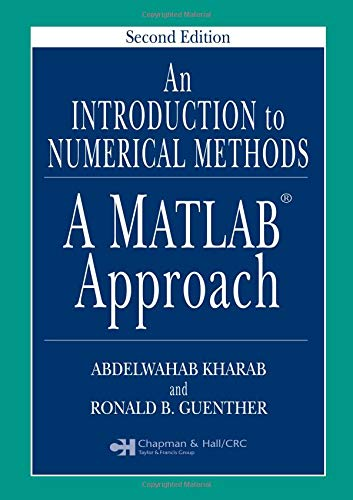# An Introduction to Numerical Methods: A MATLAB Approach, Second Edition

## Kharab, Abdelwahab; Guenther, Ronald B.

Valutazione media 4,38
( su 8 valutazioni fornite da Goodreads )Vedi tutte le copie di questo ISBN:

Numerical methods are a mainstay of researchers and professionals across the many mathematics, scientific, and engineering disciplines. The importance of these methods combined with the power and availability of today's computers virtually demand that students in these fields be well versed not only in the numerical techniques, but also in the use of a modern computational software package.

Updated to reflect the latest version of MATLAB, the second edition of An Introduction to Numerical Methods continues to fulfill both these needs. It introduces the theory and applications of the most commonly used techniques for solving numerical problems on a computer. It covers a wide range of useful algorithms, each presented with full details so that readers can visualize and interpret each step.

Highlights of the second edition:

• A new chapter on numerical optimization
• New sections on finite elements
• More exercises and applied problems in each chapter
• MATLAB incorporated as an integral part of the text

Emphasis on understanding how the methods work, a simple, direct style, and thorough coverage make this book an outstanding initiation that allows students to see almost immediate results. It will boost their confidence in their ability to master the subject and give them valuable experience in the use of MATLAB.
• Le informazioni nella sezione "Riassunto" possono far riferimento a edizioni diverse di questo titolo.

Contenuti:

INTRODUCTION
An Introduction to MATLAB
Taylor Series
NUMBER SYSTEM AND ERRORS
Floating-Point Arithmetic
Round-Off Errors
Truncation Error
Interval Arithmetic
ROOTS OF EQUATIONS
The Bisection Method
The Method of False Position
Fixed-Point Iteration
The Secant method
Newton's Method
Convergence of the Newton and Secant Methods
Multiple Roots and the Modified Newton Method
Newton's Method for Nonlinear Systems
SYSTEM OF LINEAR EQUATIONS
Matrices and Matrix Operations
Naïve Gaussian Elimination
Gaussian Elimination with Scaled Partial Pivoting
LU Decomposition
Iterative Methods
INTERPOLATION
Polynomial Interpolation Theory
Newton's Divided Difference Interpolating Polynomial
The Error of the Interpolating Polynomial
Lagrange Interpolating Polynomial
INTERPOLATION WITH SPLINE FUNCTIONS
Piecewise Linear Interpolation
Natural Cubic Splines
THE METHOD OF LEAST SQUARES
Linear Least Squares
Least Squares Polynomial
Nonlinear Least Squares
Trigonometric Least Squares Polynomial
NUMERICAL OPTIMIZATION
Analysis of Single-Variable Functions
Line Search Methods
Minimization Using Derivatives
NUMERICAL DIFFERENTIATION
Numerical Differentiation
Richardson's Formula
NUMERICAL INTEGRATION
Trapezoidal Rule
Simpson's Rule
Romberg Algorithm
NUMERICAL METHODS FOR DIFFERENTIAL EQUATIONS
Euler's Method
Error Analysis
Higher Order Taylor Series Methods
Runge-Kutta Methods
Multistep Methods
Predictor-Corrector Methods
Numerical Stability
Higher Order Equations and Systems of Differential Equations
Implicit Methods and Stiff Systems
Phase Plane Analysis: Chaotic Differential Equations
BOUNDARY-VALUE PROBLEMS
Finite-Difference Methods
Shooting Methods
EIGENVALUES AND EIGENVECTORS
Basic Theory
The Power Method
Eigenvalues for Boundary-Value Problems
Bifurcations in Differential Equations
PARTIAL DIFFERENTIAL EQUATIONS
Parabolic Equations
Hyperbolic Equations
Elliptic Equations
Introduction to Finite Element Method
Bibliography and References
Appendices
Calculus Review
MATLAB Built-in Functions
Text MATLAB Functions
Index

Each chapter also contains a section of Applied Problems.

Product Description:

Book by Kharab Abdelwahab Guenther Ronald B

Le informazioni nella sezione "Su questo libro" possono far riferimento a edizioni diverse di questo titolo.

## 1.WIRELESS AD HOC NETWORKING: PERSONAL-AREA, LOCAL-AREA, AND THE SENSORY-AREA NETWORKS

ISBN 10: 1584885572 ISBN 13: 9781584885573
Nuovo Brossura Quantit�: 2
Edizione Internazionale
Da